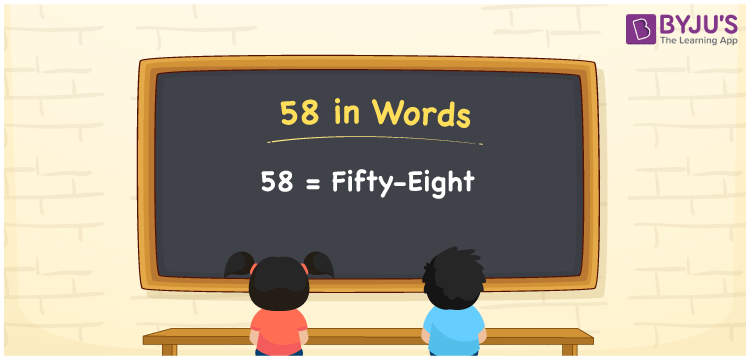# 58 in Words

58 in words is Fifty-Eight. The transformation of the number 58 into words is easy as it is a two-digit number. If you got 58 rupees as a prize in a game, then you can say, “I got Rupees 58 as a prize in a game”. 58 is a cardinal number as it represents a certain value. In this article, let us learn the spelling of 58 in English.

 58 in Words Fifty-Eight Fifty-Eight in numerical form 58

## 58 in English Words

We can convert the numbers in words using the English alphabet. Thus, in English, the number 58 is spelt as Fifty-Eight.## How to Write 58 in Words?

58 is a two-digit number. So, let us create a place value chart of two columns as represented below.

 Tens Ones 5 8

Therefore, we can write this in expanded form as:

5 x Ten + 8 x One

= 5 x 10 + 8 x 1

= 50 + 8

= 58

= Fifty-Eight

Hence, 58 in words is written as Fifty-Eight

Interesting way of writing 58 in words

5 = Five

8 = Eight

50 = Fifty

50 + 8 = 58

Fifty + Eight = Fifty-Eight

Thus, the word form of the number 58 is Fifty-Eight.

58 is a natural number that is the successor of 57 and the predecessor of 59

• 58 in words – Fifty-Eight
• Is 58 an odd number? – No
• Is 58 an even number? – Yes
• Is 58 a perfect square number? – No
• Is 58 a perfect cube number? – No
• Is 58 a prime number? – No
• Is 58 a composite number? – Yes

## Frequently Asked Questions on 58 in Words

Q1

### Express 58 in words.

We can express 58 in words as Fifty-Eight.
Q2

### What is the value of 38 + 20 in words?

38 + 20 = 58 Thus, 58 in words is Fifty-Eight.
Q3

### 58 is an even number. True or False.

True, 58 is an even number.